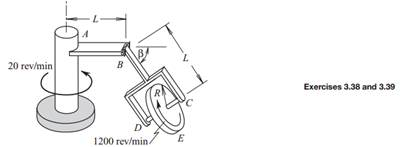### Create an Account

Home / Questions / The disk spins about its axis CD at 1200 revmin as the system rotates about the vertical a...

# The disk spins about its axis CD at 1200 revmin as the system rotates about the vertical axis at 20 revmin Both rates are constant It is desired to determine the velocity and acceleration of point

The disk spins about its axis CD at 1200 rev/min as the system rotates about the vertical axis at 20 rev/min. Both rates are constant. It is desired to determine the velocity and acceleration of point E, which is the lowest point on the perimeter of the disk, in the situation in which β is constant at 60°.

(a) Carry out the analysis by using a moving reference frame attached to gimbal BCD.

(b) Carry out the analysis by using a moving reference frame attached to the disk.Jun 16 2020 View more View LessSubscribe To Get Solution